### Home > A2C > Chapter 13 > Lesson 13.1.2 > Problem13-30

13-30.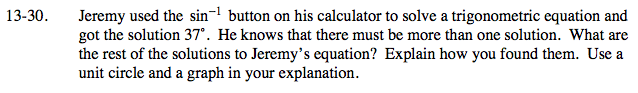Using a unit circle:

Draw a Unit circle and estimate 37 degrees.

Since sin is height, draw in the height to the x-axis.

Find and draw the same height any other place it may occur on the unit circle.

Calculate the new angles.

180° − 37° = 143°, so 37 + 2πn and 143 + 2πn where n is any integer.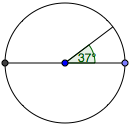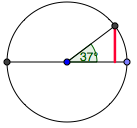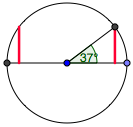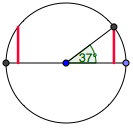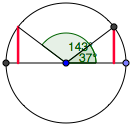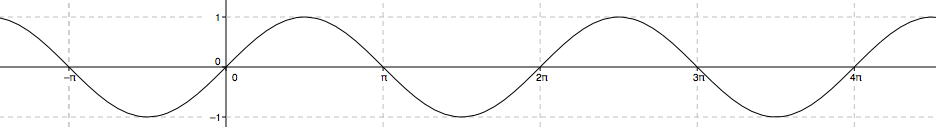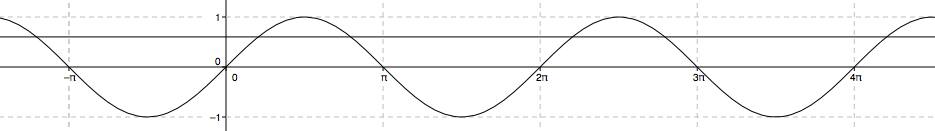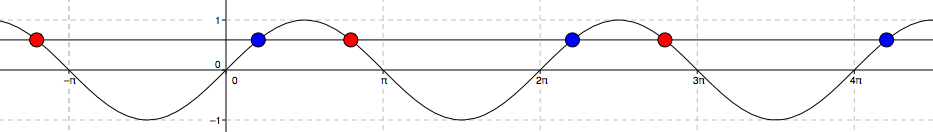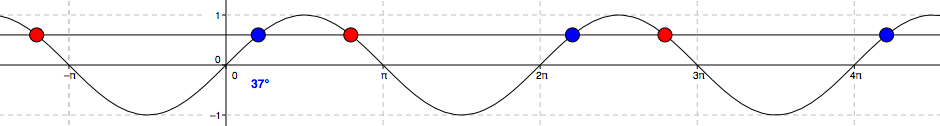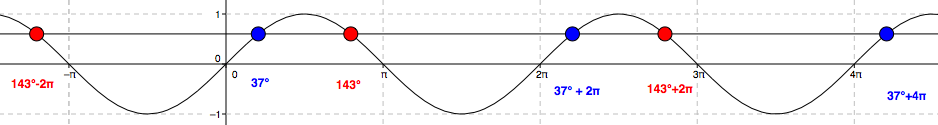Using a graph:

Draw the sine curve.

Draw y = sin(37)

Find the x-values where the two graphs intersect.

Label the solution 37°.

Use Symmetry to find other solutions.